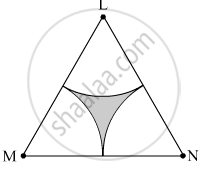# Δ Lmn is an Equilateral Triangle. Lm = 14 Cm. as Shown in Figure, Three Sectors Are Drawn with Vertices as Centres and Radius 7 Cm. Find, a ( δ Lmn) - Geometry

Sum
$∆$ LMN is an equilateral triangle. LM = 14 cm. As shown in the figure, three sectors are drawn with vertices as centers and radius 7 cm.
Find, A ( $∆$ LMN)#### Solution

∆LMN is an equilateral triangle.
∴ LM = MN = LN = 14 cm
∠L = ∠M = ∠N = 90º  Area of ∆LMN = $\frac{\sqrt{3}}{4} \left( \text{ Side} \right)^2 = \frac{\sqrt{3}}{4} \times \left( 14 \right)^2 = \frac{1 . 732}{4} \times 196$ =84.87 cm2

Concept: Areas of Sector and Segment of a Circle
Is there an error in this question or solution?

#### APPEARS IN

Balbharati Mathematics 2 Geometry 10th Standard SSC Maharashtra State Board
Chapter 7 Mensuration
Practice set 7.3 | Q 13.1 | Page 155